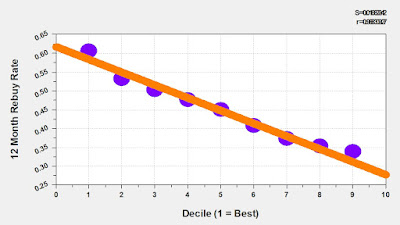## March 13, 2018

### The LTV of an Item

Here's a common scenario. Back in 2013, you introduced 800 new items. Here is the annual demand from the items.

• 2013 = \$15,300,000.
• 2014 = \$17,600,000.
• 2015 = \$9,500,000.
• 2016 = \$5,800,000.
• 2017 = \$3,400,000.
Let's assume that the items have a 55% gross margin. Here's your gross margin dollars.
• 2013 = \$8,415,000.
• 2014 = \$9,680,000.
• 2015 = \$5,225,000.
• 2016 = \$3,190,000.
• 2017 = \$1,870,000.
Each year has a "rate of dropoff".
• 2014 = 1.15.
• 2015 = 0.54.
• 2016 = 0.61.
• 2017 = 0.59.
If we assume that the dropoff will be 58% for each of the next three years, we can "guesstimate" gross margin dollars for 2018, 2019, and 2020.

• 2013 = \$8,415,000.
• 2014 = \$9,680,000.
• 2015 = \$5,225,000.
• 2016 = \$3,190,000.
• 2017 = \$1,870,000.
• 2018 = \$1,085,000.
• 2019 =    \$629,000.
• 2020 =    \$365,000.
Let's sum gross margin dollars from 2013 projected through 2020, for the Class of 2013.
• Total Gross Margin Dollars = \$30,459,000.
• New Items in 2013 = 800.
• Gross Margin Dollars per Item = \$38,074.
Technically, you could back out variable operating expenses and ad costs and get down to variable profit if you like ... have at it!  But this should prove the point ... each item has long-term value that can easily be quantified.

And if your merchandising team wants to cut back on new items by 100 next year, you can tell them that they're costing the company \$3.8 million in gross margin over the next eight years, with more than half coming in the next two years. Yup, that's \$2,000,000 of gross margin over the next two years flushed down the toilet.

Spend time taking your customer analytics skills to the merchandising realm. You're going to find that this is "fertile ground" for improvement ... under-served by the vendor community but fully appreciated by your CFO and CEO.